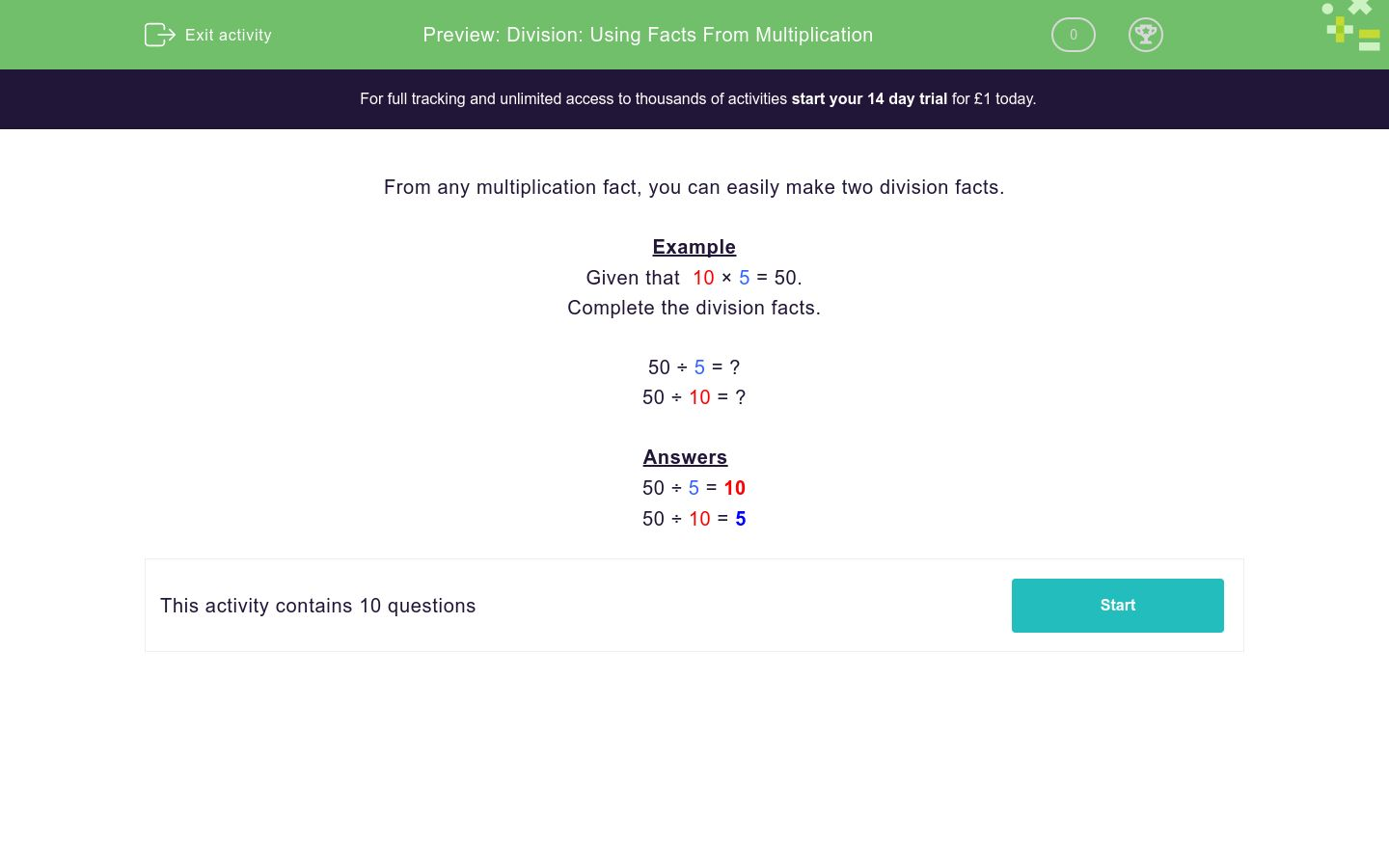# Division: Using Facts From Multiplication

In this worksheet, students learn to write down two simple division facts from a multiplication fact.Key stage:  KS 2

Curriculum topic:   Number: Multiplication and Division

Curriculum subtopic:   Write Multiplication/Division Statements for Known Tables

Difficulty level:### QUESTION 1 of 10

From any multiplication fact, you can easily make two division facts.

Example

Given that  10 × 5 = 50.

Complete the division facts.

50 ÷ 5 = ?

50 ÷ 10 = ?

50 ÷ 5 = 10

50 ÷ 10 = 5

Given that 7 × 2 = 14, complete the division facts.

14 ÷ 2 = ?

14 ÷ 7 = ?

 Answer 14 ÷ 2 14 ÷ 7

Given that 5 × 2 = 10, complete the division facts.

10 ÷ 2 = ?

10 ÷ 5 = ?

 Answer 10 ÷ 2 10 ÷ 5

Given that 8 × 10 = 80, complete the division facts.

80 ÷ 10 = ?

80 ÷ 8 = ?

 Answer 80 ÷ 10 80 ÷ 8

Given that  4 × 5 = 20, complete the division facts.

20 ÷ 5 = ?

20 ÷ 4 = ?

 Answer 20 ÷ 5 20 ÷ 4

Given that  5 × 10 = 50, complete the division facts.

50 ÷ 10 = ?

50 ÷ 5 = ?

 Answer 50 ÷ 10 50 ÷ 5

Given that  11 × 5 = 55, complete the division facts.

55 ÷ 5 = ?

55 ÷ 11 = ?

 Answer 55 ÷ 5 55 ÷ 11

Given that  1 × 5 = 5, complete the division facts.

5 ÷ 5 = ?

5 ÷ 1 = ?

 Answer 5 ÷ 5 5 ÷ 1

Given that  7 × 10 = 70, complete the division facts.

70 ÷ 10 = ?

70 ÷ 7 = ?

 Answer 70 ÷ 10 70 ÷ 7

Given that  6 × 5 = 30, complete the division facts.

30 ÷ 5 = ?

30 ÷ 6 = ?

 Answer 30 ÷ 5 30 ÷ 6

Given that  19 × 12 = 228, complete the division facts.

228 ÷ 12 = ?

228 ÷ 19 = ?

 Answer 228 ÷ 12 228 ÷ 19
• Question 1

Given that 7 × 2 = 14, complete the division facts.

14 ÷ 2 = ?

14 ÷ 7 = ?

 Answer 14 ÷ 2 14 ÷ 7
• Question 2

Given that 5 × 2 = 10, complete the division facts.

10 ÷ 2 = ?

10 ÷ 5 = ?

 Answer 10 ÷ 2 10 ÷ 5
• Question 3

Given that 8 × 10 = 80, complete the division facts.

80 ÷ 10 = ?

80 ÷ 8 = ?

 Answer 80 ÷ 10 80 ÷ 8
• Question 4

Given that  4 × 5 = 20, complete the division facts.

20 ÷ 5 = ?

20 ÷ 4 = ?

 Answer 20 ÷ 5 20 ÷ 4
• Question 5

Given that  5 × 10 = 50, complete the division facts.

50 ÷ 10 = ?

50 ÷ 5 = ?

 Answer 50 ÷ 10 50 ÷ 5
• Question 6

Given that  11 × 5 = 55, complete the division facts.

55 ÷ 5 = ?

55 ÷ 11 = ?

 Answer 55 ÷ 5 55 ÷ 11
• Question 7

Given that  1 × 5 = 5, complete the division facts.

5 ÷ 5 = ?

5 ÷ 1 = ?

 Answer 5 ÷ 5 5 ÷ 1
• Question 8

Given that  7 × 10 = 70, complete the division facts.

70 ÷ 10 = ?

70 ÷ 7 = ?

 Answer 70 ÷ 10 70 ÷ 7
• Question 9

Given that  6 × 5 = 30, complete the division facts.

30 ÷ 5 = ?

30 ÷ 6 = ?

 Answer 30 ÷ 5 30 ÷ 6
• Question 10

Given that  19 × 12 = 228, complete the division facts.

228 ÷ 12 = ?

228 ÷ 19 = ?

 Answer 228 ÷ 12 228 ÷ 19
---- OR ----

Sign up for a £1 trial so you can track and measure your child's progress on this activity.

### What is EdPlace?

We're your National Curriculum aligned online education content provider helping each child succeed in English, maths and science from year 1 to GCSE. With an EdPlace account you’ll be able to track and measure progress, helping each child achieve their best. We build confidence and attainment by personalising each child’s learning at a level that suits them.

Get started